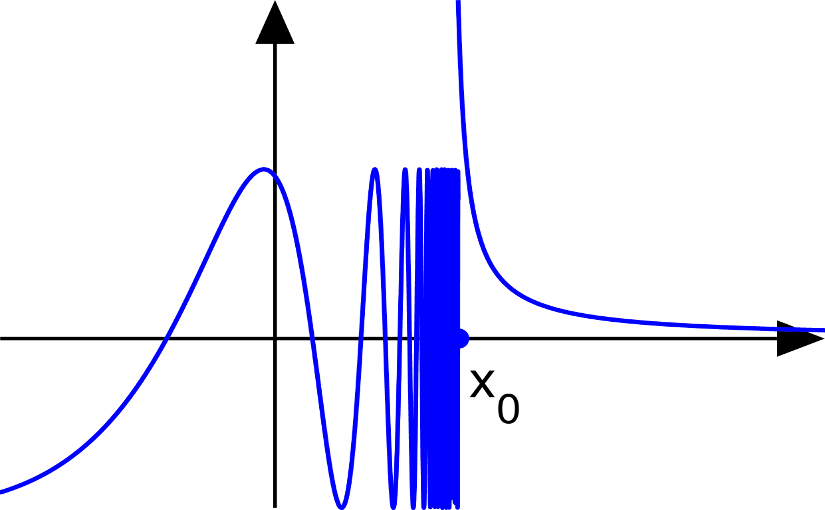# cs193p – Project #3 Assignment #3 Task #9Please note, this blog entry is from a previous course. You might want to check out the current one.

Your graphing calculator must be able to graph discontinuous functions properly (i.e. it must only draw lines to or from points which, for a given value of M, the program being graphed evaluates to a Double (i.e. not nil) that .isNormal or .isZero).

Part of this task we have already handled in the previous one, not drawing when the data source returns zero or “not normal” values. But e.g. for 1/x we still had a line from negative infinity to positive infinity. To avoid this move to new points instead of drawing lines there, when we had “impossible” previous values:

```    override func drawRect(rect: CGRect) {
...
if let y = dataSource?.y((point.x - origin.x) / scale) {
if !y.isNormal && !y.isZero {
firstValue = true
continue
}
...
} else {
firstValue = true
}
...
}
```

The complete code for the task #9 is available on GitHub.

## 3 thoughts on “cs193p – Project #3 Assignment #3 Task #9”

1. Darren Ortiz says:

This doesn’t seem to actually work. If you try and graph 1/x it still connects the line at the two extremes because the value returned from the y(x) method is always either zero or normal. It’s hard to see because the connection is being drawn right on top of the y axis, but if you try this function, it’ll be more obvious: 1 / (M – 1). I haven’t found a way to solve this yet.

1. Arjan says:

My implementation to solve that:

Instead of just continuing and adding a line, I move to the next point without drawing the line.

var path = UIBezierPath()
var onlyMove = true
for var pixelX = (bounds.minX * contentScaleFactor); pixelX <= (bounds.maxX * contentScaleFactor); pixelX++ {

let pointX = CGFloat(pixelX) / contentScaleFactor
var currentXInGraph = (pointX – origin!.x) / scale

let first = pixelX == bounds.minX

if let currentYInGraph = source.yForX(currentXInGraph) {
if currentYInGraph.isNormal || currentYInGraph.isZero {

let pointY = origin!.y – (currentYInGraph * scale)

let next = CGPointMake(pointX, pointY)
if first || onlyMove {
path.moveToPoint(next)
} else {
}
onlyMove = false
} else {
onlyMove = true
}
}
}

2. Duncan says:

Thanks for posting all of this! As someone doing CS 193P self paced via iTunes U, it’s really helpful to have this material available for help when I’m stuck!

This solution works for certain values of ‘scale’. But after zooming in or out on the graph ‘scale’ can end up as a longer decimal value. In the example of 1 / (M – 1), you can get into a situation where ‘currentXInGraph’ never hits the undefined value, like so:
x = .9674
x = .9934
x = 1.0192

In this scenario, the drawRect will end up connecting the point at x = .9934 to the point at x = 1.0192, since it never evaluated y at x = 1. Do you have any ideas on how to solve that?